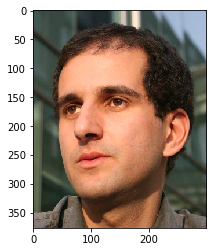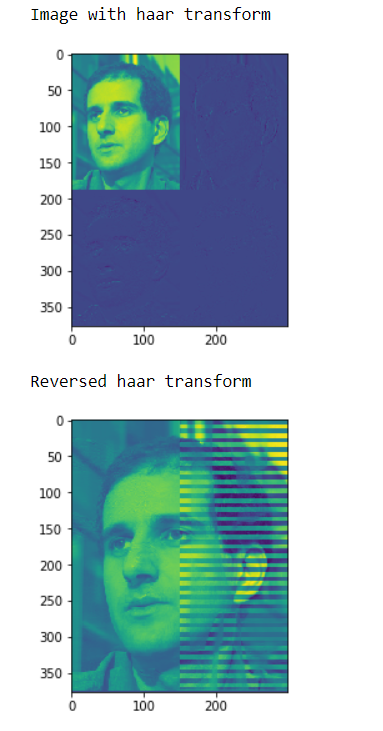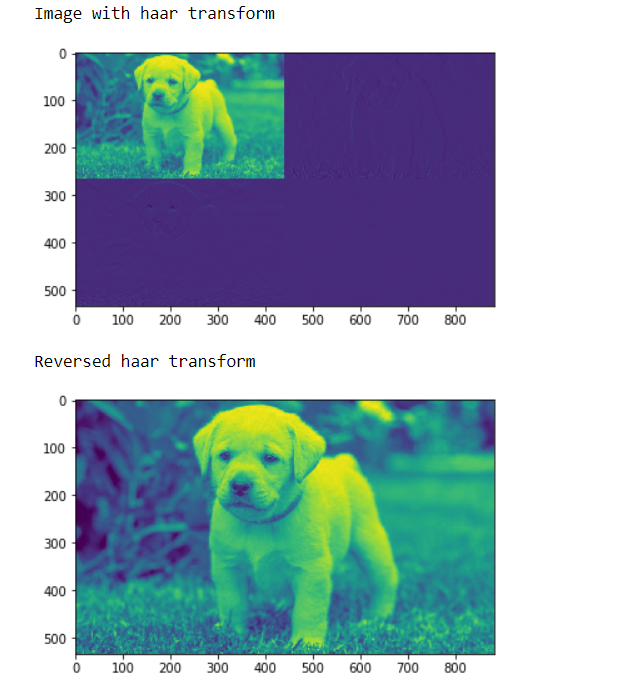# Mahotas – Reversing Haar Transform

In this article we will see how we can reverse image haar transform in mahotas. The haar wavelet is a sequence of rescaled “square-shaped” functions which together form a wavelet family or basis. Wavelet analysis is similar to Fourier analysis in that it allows a target function over an interval to be represented in terms of an orthonormal basis. The Haar sequence is now recognised as the first known wavelet basis and extensively used as a teaching example. We can do haar transform with the help of mahotas.haar method
In this tutorial we will use “luispedro” image, below is the command to load it.

`mahotas.demos.load('luispedro')`

Below is the luispedro imageIn order to do this we will use mahotas.ihaar method

Syntax : mahotas.ihaar(haar_img)
Argument : It takes image object as argument
Return : It returns image object

Note : Input image should be filtered or should be loaded as grey
In order to filter the image we will take the image object which is numpy.ndarray and filter it with the help of indexing, below is the command to do this

`image = image[:, :, 0]`

Example 1:

## Python3

 `# importing various libraries` `import` `numpy as np` `import` `mahotas` `import` `mahotas.demos` `from` `mahotas.thresholding ``import` `soft_threshold` `from` `pylab ``import` `imshow, show` `from` `os ``import` `path`   `# loading image` `f ``=` `mahotas.demos.load(``'luispedro'``, as_grey ``=` `True``)`   `# haar transform` `h ``=` `mahotas.haar(f)`   `# showing image` `print``("Image with haar transform")` `imshow(h)` `show()`   `# reversing haar transform` `r ``=` `mahotas.ihaar(h)`   `# showing image` `print``("``Reversed` `haar transform")` `imshow(r)` `show()`

Output :Example 2:

## Python3

 `# importing required libraries` `import` `mahotas` `import` `numpy as np` `from` `pylab ``import` `imshow, show` `import` `os`     `# loading image` `img ``=` `mahotas.imread(``'dog_image.png'``)`   `# filtering image` `img ``=` `img[:, :, ``0``]`     `# haar transform` `h ``=` `mahotas.haar(img)`   `# showing image` `print``("Image with haar transform")` `imshow(h)` `show()`   `# reversing haar transform` `r ``=` `mahotas.ihaar(h)`   `# showing image` `print``("``Reversed` `haar transform")` `imshow(r)` `show()`

Output :Whether you're preparing for your first job interview or aiming to upskill in this ever-evolving tech landscape, GeeksforGeeks Courses are your key to success. We provide top-quality content at affordable prices, all geared towards accelerating your growth in a time-bound manner. Join the millions we've already empowered, and we're here to do the same for you. Don't miss out - check it out now!

Previous
Next